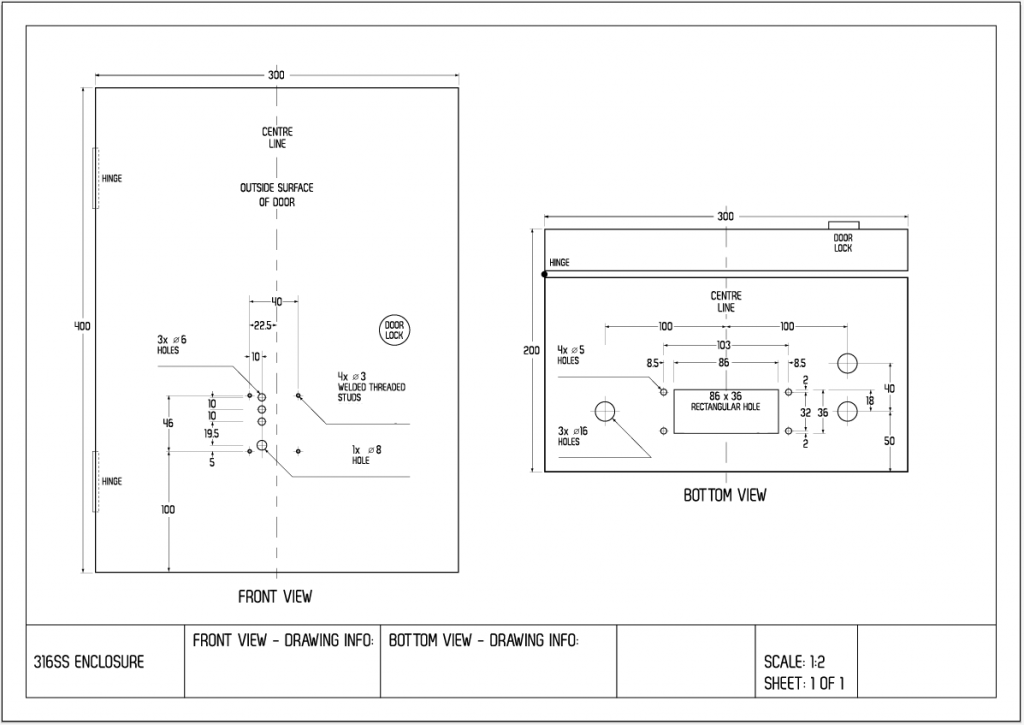1800 367 765
+61 7 3054 5900

Installation & Service Technician

February 2019

Thank you for your interest in the role of Installation & Service Technician.

Applicants must complete a 2-Step process:

Step 1: Complete an online quiz to assess your suitability for the roleStep 2: Email your resume and cover letter* to [email protected]

*cover letter is optional

Step 1: Complete the online quiz

Please complete the applicant quiz. The quiz involves 3 sections to assess your suitability for the role including:

• Numeracy Test
• Literacy Test
• Spatial Test

You will have a total of 45 minutes to complete the quiz. You will have one attempt to complete the quiz.

Hint: it should take about 10 minutes for each section. There is only one correct answer for each question.

Good luck!

Begin quiz here.
Please scroll to the top and bottom of each page to ensure you answer all questions.

Name
Email
Phone
1. What units would be best to use to measure the weight (mass) of an egg?
2. Which one of the following numbers is between 0.01 and 0.02?
3. If there are 300 calories in 100g of a certain food, how many calories are there in a 30g portion of this food?
4. -2 x 4 =
5. The simplest form of 13/6 is
6. What is 30% of 100?
7. From the graph below, which segment represents 25%?8. A flavoured cordial drink is in the ratio 1:4. How many millilitres of water will a 200ml drink contain?
9. The temperature reading on the thermometer, accurate to the nearest degree in degrees Celsius is: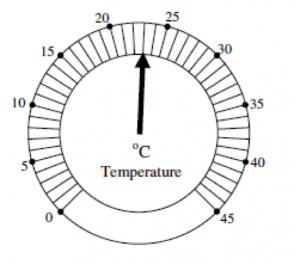10. A patient had a pulse rate of 98, 96, 97 and 93 on four occasions. The patient's average (mean) pulse rate was:
11. An object weighs 8 grams and has a volume of 5 cubic centimetres. What is the object’s density in grams per cubic centimetre (g/cm3)?
12. Select the word or phrase that correctly completes the sentence in brackets [...]. The nurse left the patient records […] the hospital.
13. Which sentence is correctly completed by the word [angel]?
14. Select the word in [brackets] that is spelt incorrectly. The person [desided] to put all her [clothes] in the bottom [drawer] of the [cupboard] next to the [window].
15. Which word has the same meaning as the word that is in [brackets]? The person is [seriously] ill.
16. Which sound [in brackets] is the same as the underlined sound [in brackets] in the following word? sh[o]ne

For questions 17 to 20, please refer to the following article.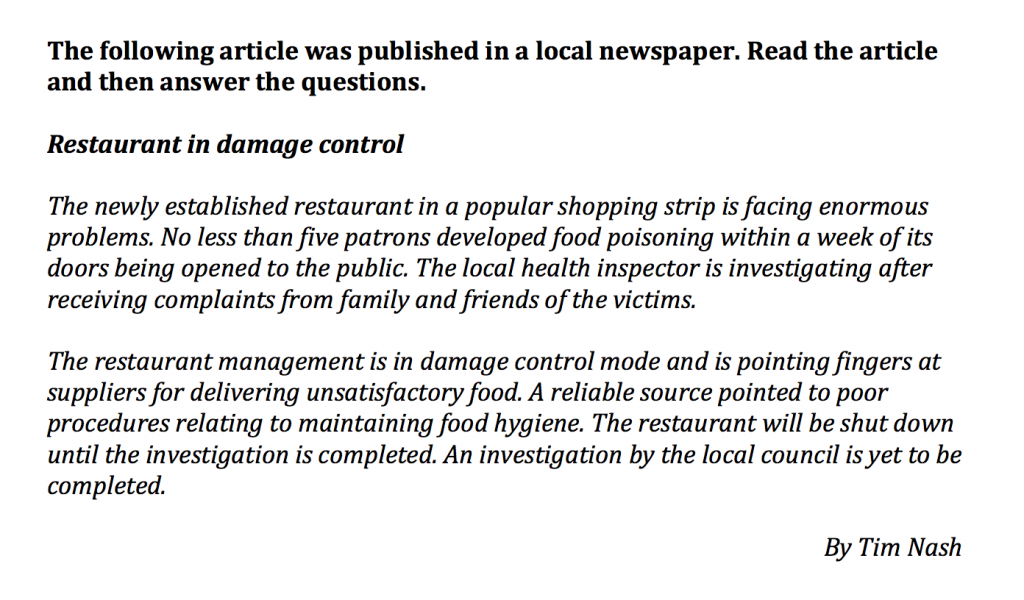17. Read the article and then answer the questions. Who is investigating?

18. Read the article and then answer the questions. The source who mentioned a possible reason is [...]?

19. Read the article and then answer the questions. The restaurant will be shut down until [...]?

20. Read the article and then answer the questions. The food delivered by the suppliers is unsatisfactory because it may be [...]?

21. In the figure shown below, one of the shapes (A-D) is identical to the first figure but has been rotated. Which figure is identical to the first?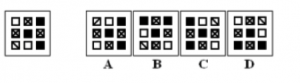22. In the figure shown below, one of the shapes (A-D) is identical to the first figure but has been rotated. Which figure is identical to the first?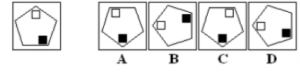23. In the figure shown below, one of the shapes (A-D) is identical to the first figure but has been rotated. Which figure is identical to the first?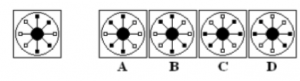24. In the figure shown below, one of the shapes (A-D) is identical to the first figure but has been rotated. Which figure is identical to the first?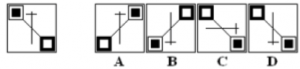25. In the figure shown below, one of the shapes (A-D) is identical to the first figure but has been rotated. Which figure is identical to the first?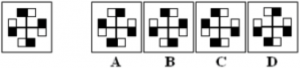26. Which pattern can be folded to make the cube shown?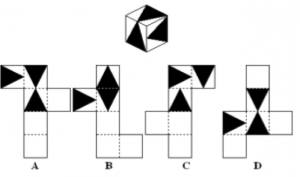27. Which pattern can be folded to make the cube shown?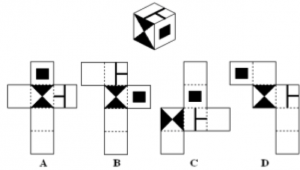28. Which pattern can be folded to make the cube shown?29. Which pattern can be folded to make the cube shown?30. In the following drawing, what are the outside dimensions (Width x Height x Depth) of the Enclosure?